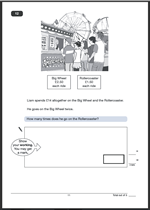# KS2 Maths Paper 2010 Question 12This is a typical SAT question, which many children fail to get correct. The main reason for this is that it is in three parts and many children cannot progress through a step by step approach, wanting to find the answer in one easy move. The second reason is that it involves dividing by a decimal fraction at the end.

The question asks how many times Liam goes on the Rollercoaster, with each ride costing £1.50.

Begin by finding how much two goes on the big wheel costs as this will show how much he has spent before going on the Rollercoaster.

That’s 2 x £2.50 which is £5.

Now to find how much he has left to spend. Subtract the £5 from the total spent.
That’s £14 – £5 = £9
Then work out how many times Liam could go on the Rollercoaster with £9. Probably the easiest way to do this is to work out that two rides would cost £3, so 6 rides would cost £9. Now, whilst the calculation might have been done mentally it is important to write down what was done.

Two marks for a correct answer, but one mark can be given if the correct method is shown.

Question 12 from SATS Paper A 2010

Question 12 answer and suggested method.